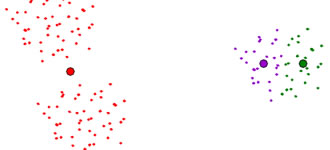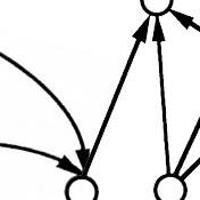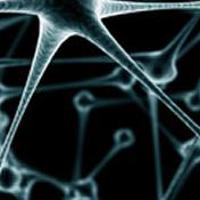## K-Means clustering

K-Means is a clustering algorithm. That means you can "group" points based on their neighbourhood. When a lot of points a near by, you mark them as one cluster. With K-means, you can find good center points for these clusters.

This "clustering" is not limited to two dimensions. You can have points in 3 dimensions. Or any number of dimensions you want.In the above image, every non-white pixel is a data point. Some experiment/algorithm/something produced them. You can see the points have been grouped into four clusters. That's "clustering" in action.

## K-means algorithm

You must supply two things to the algorithm:

• The data points themselves
• K - The number of clusters

So, you should know the number of clusters before hand. This might be a problem in some situations, but there are hacks to use K-means when you don't know the number of clusters.

After the algorithm finishes, it produces these outputs:

• A label for each data point
• The center for each label

A label can be considered as "assigning a group". For example, in the above image you can see four "labels". Each label is displayed with a different colour. All yellow points could have the label 0, orange could have label 1, etc.

Now into the algorithm.

### Step 0: Get the dataset

As an example, I'll be using the data points on the left. We'll assume K=5. And it's apparent that this dataset has 5 clusters: three spaced out and two almost merging.

### Step 1: Assign random centers

The first step is to randomly assign K centers. I've marked them as red points in the image. Note how they are all concentrated in the two "almost merging" clusters.

These centers are just an initial guess. The algorithm will iteratively correct itself. Finally, these centers will coincide with the actual center of each cluster.

### Step 3: "Own" datapoints

Each datapoint checks which center it is closest to. So, it "belongs" to that particular center. Thus, all centers "own" some number of points.

### Step 4: Shift the centers

Each center uses the points it "owns" to calculate a new center. Then, it shifts itself to that center.

If the centers actually shifted, you again go to Step 3. If not, then the centers are the final result. You proceed to the next step

### Step 5: The end!

Now that the centers do not move, you can use the centers.

Note: These centers might not be what you expect. If the initial random guess is really weird, you might end up with bad centers! (we'll see how to get over this)

## Problems & Solutions

We've encountered two problems up till now.

### Not knowing the value of K

There is no way of knowing the number of clusters with K-means. So what you can do is start with K=1. Then increase the value of K (up till a certain upper limit). Usually, the variance (the summation of square of distance from "owner" center for each point) will decrease rapidly. After a certain point, it will decrease slowly. When you see such behaviour, you know you've overshot the K-value.

If your initial guess is bad, you cannot expect the algorithm to work well. Here's a possible scenario:The best way is to run K-means on several random initial guesses. Then, pick the final centers which have the least variance.

Another trick is to pick centers in a certain manner:

1. Place the first center on a data point
2. Place the second center on a datapoint that is farthest from the first
3. Place the third center on a data point that is farthest from the first and second
4. So on...

## Summary

You learned how the K-means algorithm works. We also looked at two problems common to the K-means algorithm, and also their solutions. Hope you found this useful!

#### Related posts#### 7 unique neural network architectures